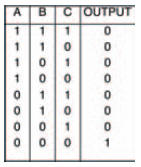# Simple, eh?Electronics guide > Digital integrated circuits I > Simple, eh?

Earlier on I mentioned that all digital circuits can be made up from the simplest of digital circuits — the inverter. I’m going to prove that now; theoretically at first, then we can do some experiments which show the fact practically.

By expanding the inverter circuit we first looked at back in Figure 10.1 and the following circuits, we can easily create other circuits. Figure 10.31, for example, is merely a transistor operating in the same way that the inverter transistor circuit of Figure 10.1 works.

It is, effectively, an inverter with three inputs to its base, rather than just one.Figure 10.31 A transistor switch, with three inputs A, B and C

If you look at it carefully, you should be able to figure out how it works. When all three of the three inputs, A, B, or C are connected to logic 0, the transistor is off, hence the transistor is a very high resistance, which means that the output is at logic 1. However, when any one of the three inputs is connected to logic 1 the transistor turns on, hence becoming a low resistance, and the output becomes logic 0. The truth table of Figure 10.32 shows this, and if you compare this to the truth table in Figure 10.25 you’ll see they are the same. Yes, the three input transistor switch of Figure 10.31 (which is merely an adapted inverter) is a NOR gate!Figure 10.32 Truth table for the transistor switch circuit of Figure 10.31

<< Other logic gates